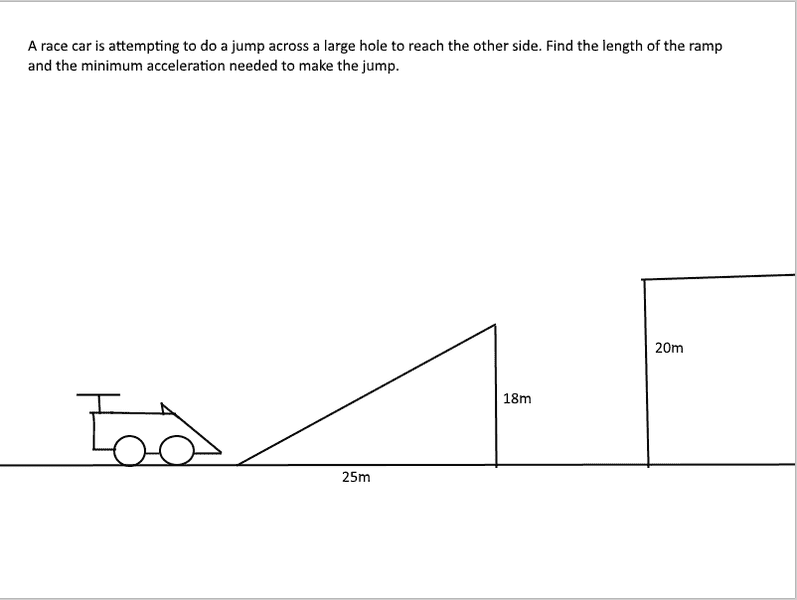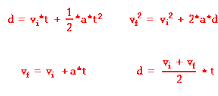# How do you find the acceleration needed to clear a jump?

## Homework Statement## Homework Equations## The Attempt at a Solution

I used Pythagorean theorem to find the length of the ramp (25^2+18^2 = 949) and found the angle of elevation using tangent (Tanθ=18/25) but then got stuck on what formula to use.

#### Attachments

RPinPA
Homework Helper
First, what velocity does it need when it leaves the ramp? Intuitively, you can see that if you leave too slowly, you're going to fall down into the gap. So what determines whether you'll make it?

The horizontal motion is constant velocity ##v \cos(\theta)##. That will determine how long it is in the air. Use the equation for d but with a = 0 since it's constant velocity. Horizontal d is fixed. You're solving for time.

The vertical motion is accelerated motion with initial vertical velocity ##v \sin(\theta)##. That will determine where the car is after that much time. Use the equation for d but with a = -9.8 m/s^2.
It will make the jump if that vertical displacement is enough. If d from where it left the ramp is enough to make it to the platform. How much higher is the platform than the end of the ramp? That's what the vertical d needs to be.

OK, now you know what the velocity needs to be at the end of the ramp. It needs to have enough acceleration to get to that velocity within the length of the ramp. Is there a formula that relates acceleration, distance and velocity? There is. Use that one, since you know two of the three variables and can solve for the other one (acceleration).

So I know the length of the ramp and the angle but how exactly do I find the velocity? For part one you mentioned using v cos(θ) to determine how long the car will be in the air but how do I use that formula if I don't know what v (assuming that v means velocity) is?

haruspex
Homework Helper
Gold Member
I don't know what v (assuming that v means velocity) is?
So leave it as unknown. You can still write the equation relating it to time of flight. Likewise for the vertical motion. Two equations, two unknowns: solve.

PeroK
Homework Helper
Gold Member
2021 Award

## Homework Statement

View attachment 230985

## Homework Equations

View attachment 230986

## The Attempt at a Solution

I used Pythagorean theorem to find the length of the ramp (25^2+18^2 = 949) and found the angle of elevation using tangent (Tanθ=18/25) but then got stuck on what formula to use.

It seems to me that you need to know the distance across the gap. Do you have that information?

•CWatters
It seems to me that you need to know the distance across the gap. Do you have that information?
nope. it would be much easier if I did but the assignment did not provide it.

So leave it as unknown. You can still write the equation relating it to time of flight. Likewise for the vertical motion. Two equations, two unknowns: solve.

So by leaving it as unknown that gives me an equation: 25=vi*t + 0. Now I don't know the time or the velocity how would I solve this?

CWatters
Homework Helper
Gold Member
Suppose the gap was 5000 km. You would have to go very fast to make the jump. I also believe it's necessary to solve the problem.

haruspex
Homework Helper
Gold Member
So by leaving it as unknown that gives me an equation: 25=vi*t + 0. Now I don't know the time or the velocity how would I solve this?
Wrong equation. How is the 25m relevant here? We want the launch speed at the top of the ramp, and the 25m is behind.
As others have noted, the question cannot be solved without knowing the width of the gap. E.g. with a gap of 2m it is impossible; no matter how fast you go you won't make it.
Let the gap width be x. If the time of flight is t and the launch speed v, what equations can you write for the horizontal and vertical displacements of the trajectory?

I see, alright thanks for your help. I it makes sense that this would be impossible since the width of the gap isn't stated so I suppose it could be any number like 5000 km or something ha ha.

haruspex